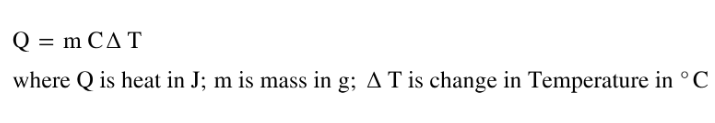# Problem: A 100.0-g bar of gold is heated from 25 oC to 50 oC, during which it absorbs 322 J of heat. Assume the volume of the gold bar remains constant.What is the molar heat capacity of Au (s) ?

###### FREE Expert Solution

Use the calorimetry equation to find specific heat of gold where100% (299 ratings)###### Problem Details

A 100.0-g bar of gold is heated from 25 oC to 50 oC, during which it absorbs 322 J of heat. Assume the volume of the gold bar remains constant.

What is the molar heat capacity of Au (s) ?

Frequently Asked Questions

What scientific concept do you need to know in order to solve this problem?

Our tutors have indicated that to solve this problem you will need to apply the Heat Capacity concept. You can view video lessons to learn Heat Capacity. Or if you need more Heat Capacity practice, you can also practice Heat Capacity practice problems.

What professor is this problem relevant for?

Based on our data, we think this problem is relevant for Professor Fakhreddine's class at TEXAS.